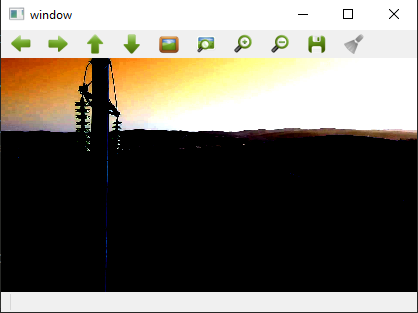# Resize images (torch.Tensor) already loaded on GPU keeping their aspect ratio

Hello everyone,

Could anyone give me a hand with the following please.
Context: I am working on a system that processed videos. I take N frames, .cat() them in a batch and move to GPU.
Then, I want to run this batch through a neural net (YOLO). In order to do it, I need to resize each image in the batch to the standard 416 x 416 size keeping the aspect ratio. The main problem here - I cannot move it back to CPU, we’re trying to minimize host-device data transfers, so it needs to be done directly on GPU.

Could anyone please explain how to do it?

What I am doing now:

1. I am looping over my torch.Tensor (batch of images on GPU) and want to resize each tensor separately. I am sure there’s a better way to do it all together.

This is obviously not working.

Eugene

You could use `F.interpolate` to reshape the tensors instead of the current copy-approach.
Let me know, if that would work for you.

Hi @ptrblck,

Thanks a lot for a quick reply.

Yes, I’ve tried this method and it is working. However, I need my image to be a square (416x416). When I use the method you suggested I am getting:If I am not mistakes, now I need to create a tensor of the shape I need and fill it with (126, 126, 126) colour and somehow combine with resized image. Is that correct? Could you please give me a hand with this or share I link where I could read how to do it?

Eugene

What kind of shape does `tensor` have and what are you passing as `new_dimension`?

Hi @ptrblck,

TENSOR SHAPE: torch.Size([5, 3, 1080, 1920]) - a batch of frames (5 in this case), rgb.
NEW DIMENSIONS: (234, 416) - recalculated frame size with the preserved aspect ratio.

No doubts you’ve already understood what I want, but just to reiterate I would love each image to be not (234, 416) but (416, 416), the rest should be filled with (126, 126, 126) colour.

Thank you

Ah, thanks for the explanation. I totally misunderstood the use case.Could you check, if this would work?

``````x = torch.randn(5, 3, 1080, 1920)
y = F.interpolate(x, (234, 416))
res = torch.ones(5, 3, 416, 416) * 126.
res[:, :, 91:325, :] = y
``````

Hi @ptrblck,

It seems to be working almost perfectly! Thank you very much. The only 2 issues is the colour background we added is white:And when I test it for tall image (height > width), it crashes with the following error:
The expanded size of the tensor (416) must match the existing size (312) at non-singleton dimension 3. Target sizes: [5, 3, 234, 416]. Tensor sizes: [3, 416, 312]

Could the output be somehow “normalized” such that the gray color would be visualized as white?
The background should have the color `[126, 126, 126]`, so I’m unsure why it’s shown as white.

I’m getting a gray background here:

``````x = torch.randint(0, 255, (5, 3, 1080, 1920)).float()
y = F.interpolate(x, (234, 416))
res = torch.ones(5, 3, 416, 416) * 126.
res[:, :, 91:325, :] = y
plt.imshow(res.permute(1, 2, 0).byte())
``````

Assuming that a “tall” image should be resizes as `[416, 234]`, you might need to change the code to:

``````if tall:
res[:, :, :, 91:325] = y
else:
res[:, :, 91:325, :] = y
``````

or

``````if tall:
idx = 3
else:
idx = 2
res.index_copy_(idx, torch.arange(91, 325), y)
``````

Hi @ptrblck,

I think you might be right, the background color should be correct. Thank you for helping with this.

I am sorry I think I failed to specify that the system can technically work with images of any size. Tall, wide, with different proportions. If I am not mistaken, the 91 and 325 numbers are hard coded for the 1080, 1920 case. Could we somehow use the new dimensions I am calculating to generalize this step, so it works with images of any random size?

UPDATE: Done. Everything’s working.

Thank you for your help and time!

Eugene

1 Like

In case anyone ever finds this thread having the same question. Here’s the working code I ended up with: